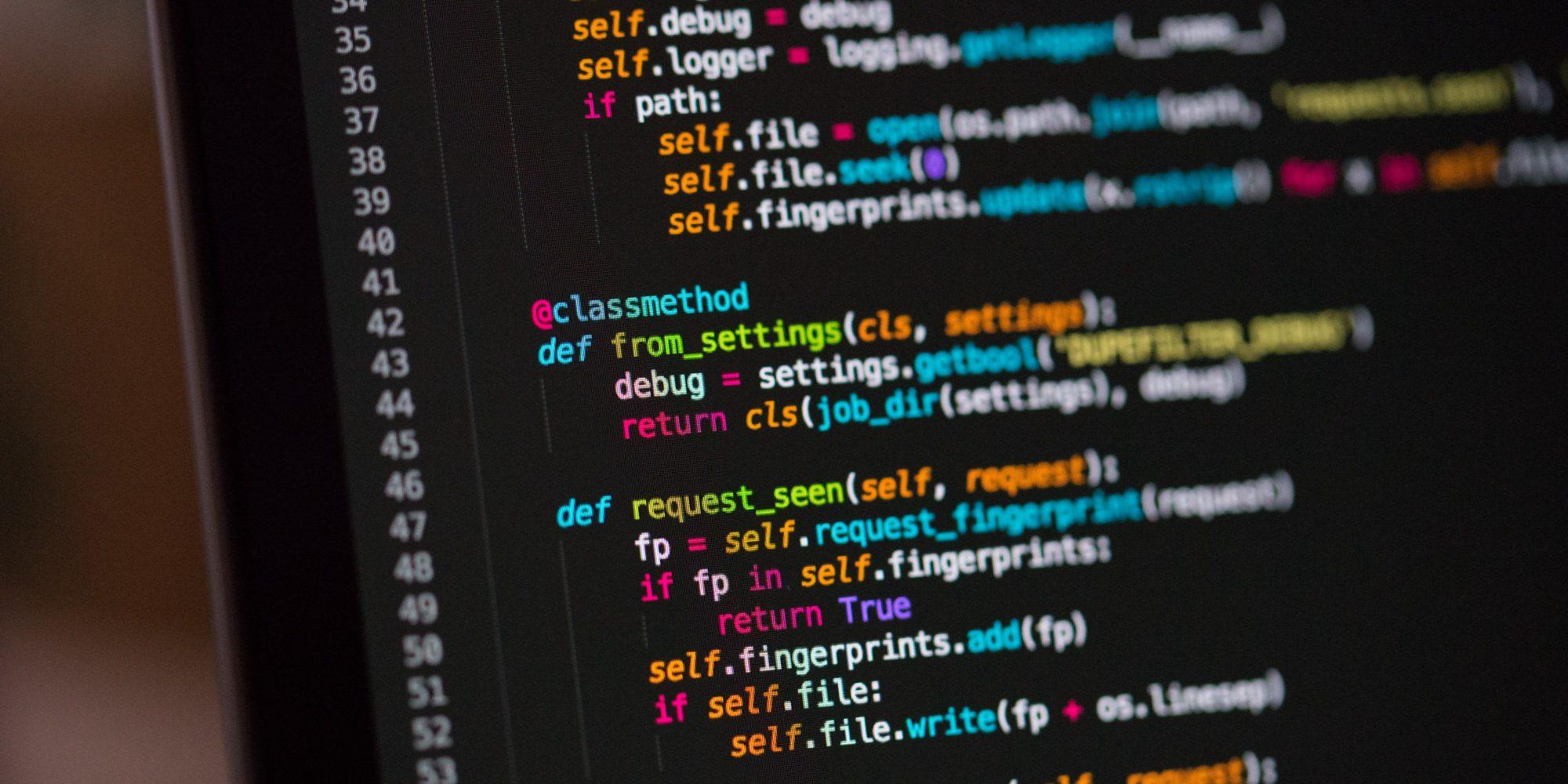# How To Round Numbers In Python

One of the best things when you are looking to round numbers is that you have many different options to do it. You can go old fashioned and do it by simply using a piece of paper and a pencil, you can use our rounding calculator, you can use Microsoft Excel, and even Python.

While for may people Python continues to be a very complicated way to round numbers, for others who love Python it is just perfect.

So, today, we are going to show you how to round numbers in Python.

## How To Round Numbers In Python

One of the things that you need to understand about Python and rounding numbers, is that there are many different rounding strategies that you can use. So, today, we are only going to look at how to round numbers in Python for rounding up.

This strategy will always round a number up to a specific number of digits.

Just take a look at the following table to see how we can summarize this rounding up strategy:

 Value Round Up To Result 12.345 Tens place 20 12.345 Ones place 13 12.345 Tenths place 12.4 12.345 Hundredths place 12.35

To use this rounding up strategy in Python, you will need to use the ceil() function from the math module.

In Python, math.ceil() implements the ceiling function and always returns the nearest integer that is greater than or equal to its input:

` >>> >>> import math >>> math.ceil(1.2) 2 >>> math.ceil(2) 2 >>> math.ceil(-0.5) 0 `

It is important to notice that the ceiling of -0.5 is 0, not -1. In case you are wondering why, the truth is that it simply makes sense since 0 is the nearest integer to -0.5 which is greater than or equal to -0.5.

You can then rewrite the function called round_up() that implements the “rounding up” strategy:

` def round_up(n, decimals=0):     multiplier = 10 ** decimals     return math.ceil(n * multiplier) / multiplier `

If you check the previous code, you can easily see that the pattern of shifting the decimal mode, applying some rounding method to round to an integer, and then shifting the decimal point back will come up over and over again as we investigate more rounding methods. And this is just the way that your brain is used to work when you need to round numbers.

One of the best things about using Python is that you can use different inputs:

`  >>> >>> round_up(1.1) 2.0 >>> round_up(1.23, 1) 1.3 >>> round_up(1.543, 2) 1.55 `

In case you prefer, just use our calculator to round numbers.

And you can even pass a negative value to decimals:

`  >>> >>> round_up(22.45, -1) 30.0 >>> round_up(1352, -2) 1400 `

If you check this passage, when you turn a negative number into decimals, the number that comes in the first argument of round_up() is rounded to the correct number of digits to the left of the decimal point.

When you pass a negative number to decimals, the number in the first argument of round_up() is rounded to the correct number of digits to the left of the decimal point.